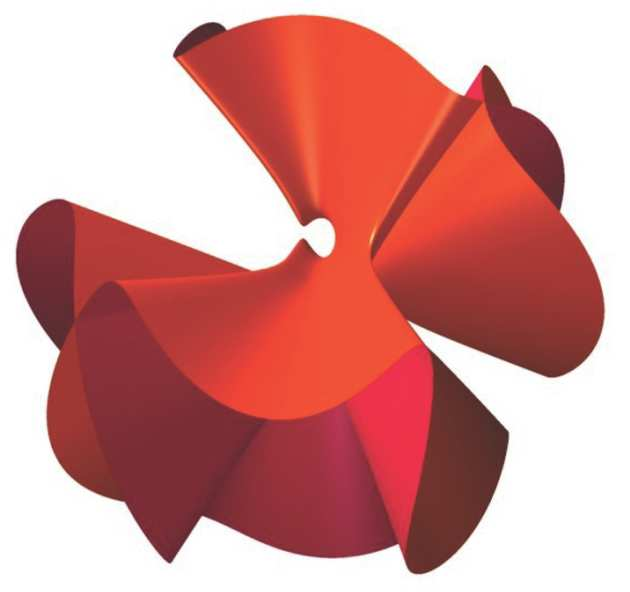# Research## Areas of research interest include:

• complex and Kähler geometry, canonical Kähler metrics
• algebraic geometry, classification theory, Mori theory, Fano varieties
• symplectic geometry
• gauge-theory, knot theory, 3- and 4- dimensional topology
• Calabi-Yau manifolds, mirror symmetry, mathematical aspects of string theory (with the theoretical physics group), derived categories
• Geometric Invariant Theory
• Gromov-Witten invariants, moduli of curves
• calibrated geometry, exceptional holonomy, special Lagrangians
• geometric analysis, nonlinear elliptic PDE, geometric flows.